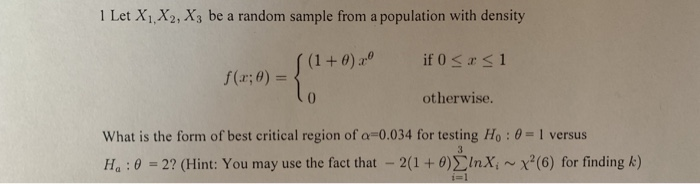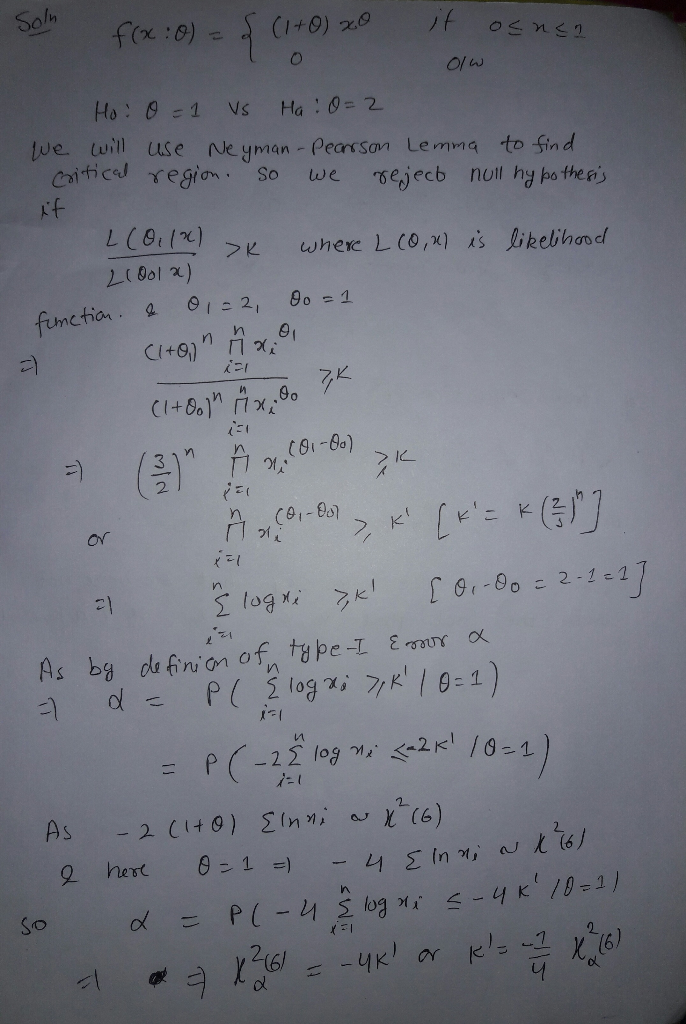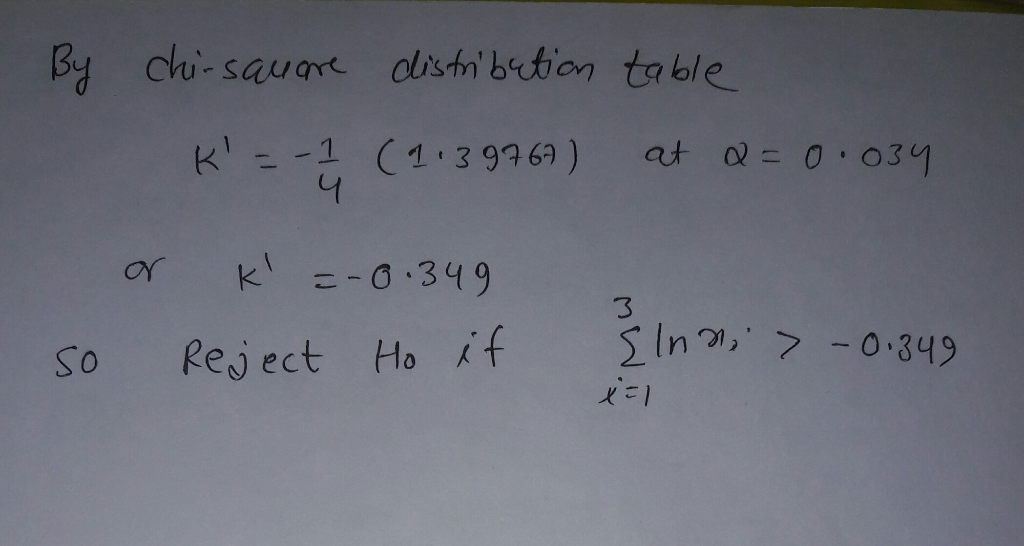Homework Help Question & Answers

# 1 Let X1, X2, X3 be a random sample from a population with density otherwise. What is the form of...1 Let X1, X2, X3 be a random sample from a population with density otherwise. What is the form of best critical region of α-0034 for testing Ho : θ 1 versus Ha : θ-27(Hint: You may use the fact that-2(1 + θ)Σ1nKi ~ χ"(6) for finding k)##### Add Answer of: 1 Let X1, X2, X3 be a random sample from a population with density otherwise. What is the form of...
More Homework Help Questions Additional questions in this topic.

• #### R and θ E (0, 1), define For x otherwise Let X, X, be i.i.d. random variables with density fo, for some unknown 0 E (0,1) 2 points possible (graded, results hidden) Let a be the number of X, which ar...

Need Online Homework Help?

Get FREE EXPERT Answers
WITHIN MINUTES
Related Questions